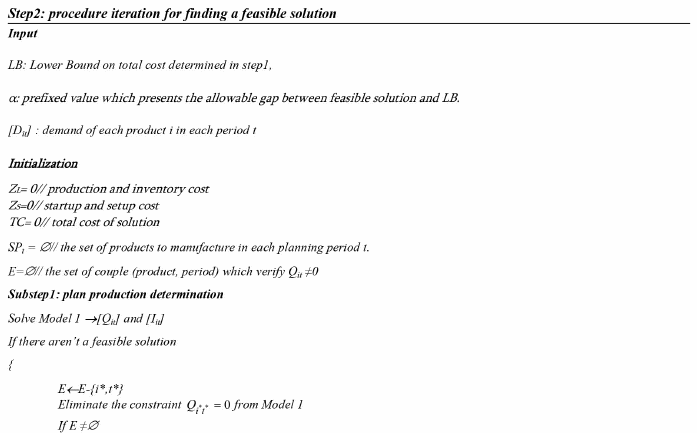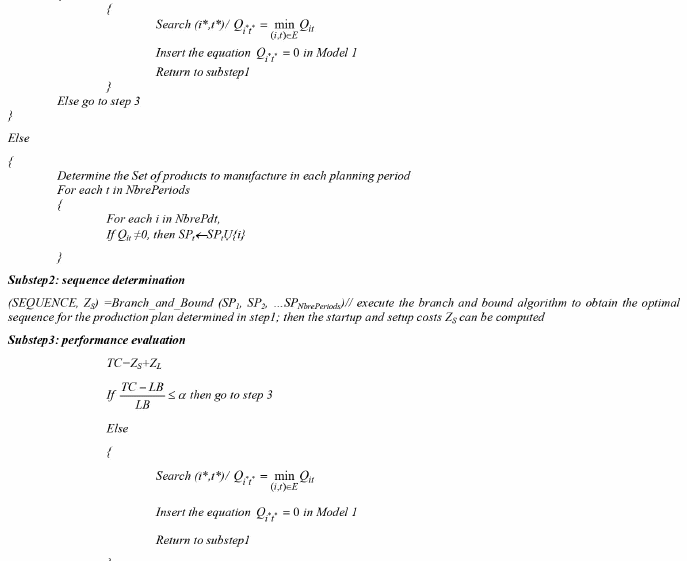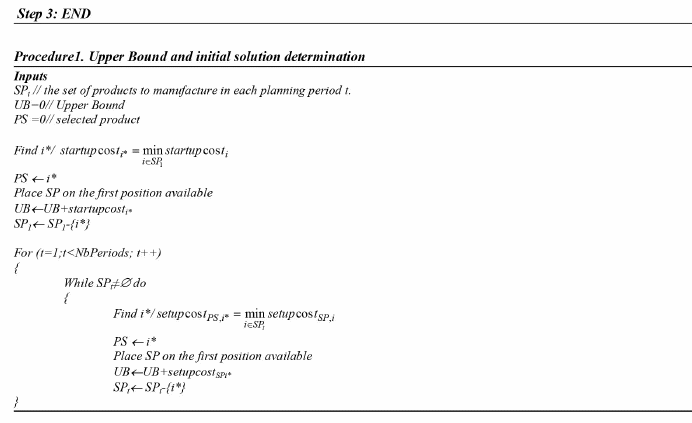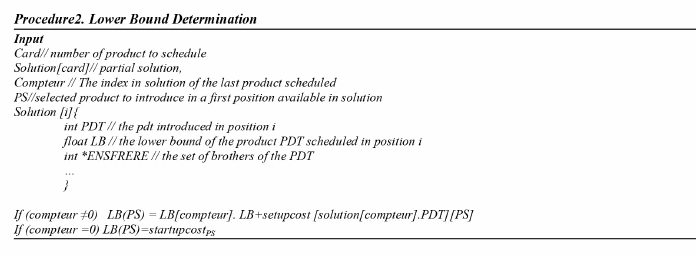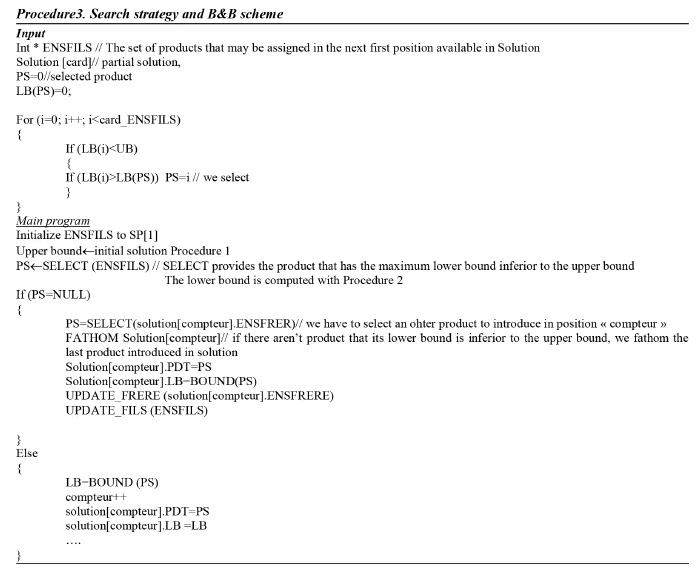A new approach for solving Capacitated Lot Sizing and scheduling Problem with Sequence and period-Dependent setup costsA new approach for solving Capacitated Lot Sizing and scheduling Problem with Sequence and period-Dependent setup costs

Imen Chaieb Memmi, Sondes Hammami Laaroussi

Ecole Supérieure de Technologie et d'Informatique (Tunisie)

Accepted: September 2013

Chaieb Memmi, I,, & Hammami Laaroussi, S. (2013). A new approach for solving Capacitated Lot Sizing and scheduling Problem with Sequence and period-Dependent setup costs. Journal of Industrial Engineering and Management, 6(4), 1027-1054. http://dx.doi.org/10.3926/jiem.707

---------------------

Abstract:

Purpose: We aim to examine the capacitated multi-item lot sizing problem which is a typical example of a large bucket model, where many different items can be produced on the same machine in one time period. We propose a new approach to determine the production sequence and lot sizes that minimize the sum of start up and setup costs, inventory and production costs over all periods.

Design/methodology/approach: The approach is composed of three steps. First, we compute a lower bound on total cost. Then we propose a three sub-steps iteration procedure. We solve optimally the lot sizing problem without considering products sequencing and their cost. Then, we determine products quantities to produce each period while minimizing the storage and variable production costs. Given the products to manufacture each period, we determine its correspondent optimal products sequencing, by using a Branch and Bound algorithm. Given the sequences of products within each period, we evaluate the total start up and setup cost. We compare then the total cost obtained to the lower bound of the total cost. If this value riches a prefixed value, we stop. Otherwise, we modify the results of lot sizing problem.

Findings: We show using an illustrative example, that the difference between the total cost and its lower bound is only 10%. This gap depends on the significance of the inventory and production costs and the machine’s capacity. Comparing the approach we develop with a traditional one, we show that we manage to reduce the total cost by 30%.

Research limitations/implications: Our model fits better to real-world situations where production systems run continuously. This model is applied for limited number of part types and periods.

Practical implications: Our approach determines the products to manufacture each time period, their economic amounts, and their scheduling within each period. This outcome should help decision makers bearing expensive start up and setup costs, to reduce their Inventory and Production costs and Start up and setup cots.

Originality/value: The main idea of the proposed approach is to intelligibly reduce the number of products to manufacture within each period in order to decrease the setup cost; since in case of limited machine’s capacity, we show that setup costs could increase when reducing the total number of parts manufactured over the entire planning horizon. In fact, the triangular inequality in setup costs is proved to be not usually available.

Keywords: multi-item capacitated lot sizing, sequence-dependent setup costs, iterative approach, production planning, scheduling

---------------------

1. Introduction

Lot sizing models are models that determine the optimal timing and the level of production. These models have to consider setup times and/or setup costs since researchers have demonstrated that to improve the production system’s performance (such by reducing makespan, improving the output capacity, reducing inventories), setup times/costs should be taken into account when determining the production planning.

The setup time is the period required to prepare a device, machine, process or system to be ready to function or accept to a job. The setup cost includes the expenses incurred in setting up a machine, a work center, or an assembly line, to switch from one production job to the next. Research by Allahverdi and Soroush (2008) display some examples of setup activities in a manufacturing system (such as obtaining tools, cleaning up), in a service organization (setting up a suitable environment to perform tasks), in a computer system (transferring programs and their dependent files) and in a synchronous circuit (holding the data signals steady). The setup cost and setup time can be proportional or not. If the resource idle time is the only considered by setting up, than the setup cost and setup time are proportional. In this case, it is sufficient to consider, either setup cost or time. In other situations, the setup time may be negligible whereas the setup cost is very important. This is the case of chemical compounds manufacturing, metal processing, food processing or paper industries, (Kolfer, Wagner, Beham, Kronberger & Affeenzeller, 2009).

Research by Karimi, Fatemi Ghomi and Wilson (2003) discern two types of setup structure: Simple and Complex. The complex setup structure can be classified into one of the following three types:

• Setup carry over: it allows continuing the production run from the previous period into the current period; and saving an additional setup cost.

• Family or major setup: applied when there are similarities in manufacturing process and design of a group of items. The family (major) setup time is incurred when at least one product of this family is produced. An individual (minor) setup time may be also incurred when a product is produced in a period.

• Sequences-dependent setup: item setup cost and time depend on the production sequence. In this case, the scheduling affect considerably the total production cost.

In case of important setup costs and/or times, it is possible that a production plan established without taking them into account is partially or totally modified when minimizing overall costs. Suppose that, according to a given production plan, product i is planned to be manufactured in period t. If the manager observes that setup costs will be considerably reduced by manufacturing i in period (t-1) and if this satisfies existing capacity restrictions, modifying the production plan will surely minimize overall costs.

Taking into account set up costs when they are sequences-dependent implies that Factory managers have to decide which products to make in which periods, and the exact production sequence and production quantities in order to minimize the sum of different costs. Thus, they have to tackle both a lot-sizing and a scheduling problem.

In this paper, we take into account these setup costs while solving a lot sizing problem. The problem we study belongs to a variety of Dynamic Lot Sizing models that takes into account these setup costs. It is called Capacitated Lot Sizing and scheduling Problem with Sequence Dependent setup costs (CLSPSD). Since this variety may not be very common, we situate it amongst the inventory models.

Among the wide variety of lot sizing problems, research by Jans and Degraeve (2007) recall that at one end of the spectrum there are the continuous time scale, constant demand and infinite time horizon lot sizing problems. In this category we find the well known Economic Order Quantity model (EOQ) and the Economic Lot Scheduling Problem (ELSP). At the other end of this spectrum we have the discrete time scale, dynamic demand and finite time horizon lot sizing models. This type of planning is generally referred to as Dynamic Lot Sizing.

Research by Ullah and Parveen (2010) give some attributes useful for distinguishing various inventory models. Among these attributes, we base on number of items to classify a single level single-resource dynamic lot sizing models into two classes:

• Single item problems: we have to decide whether to produce or not a single item in each planning period. In each period with non-zero production, a set-up is incurred. We can find the classical model ULS “Uncapacitated Lot Sizing problems”: we have to produce a single item in a single machine with unlimited capacity.

• Multi-item problems: we adopt the classification of Gupta and Magnusson (2005) and Belvaux and Wolsey (2000) based on the time window structure. We distinguish two sub classes:

• The “small buckets models” also termed “small time window” or the “Discrete Lot Sizing and scheduling problems”, it supposes that the planning horizon is divided into small time periods at which only one or two product(s) can be produced. It groups: (1) CSLP Continuous Set up Lot sizing Problem where the start up cost is introduced, (2) PLSP Proportional Lot Sizing and scheduling Problem which allows producing at most two different items in each time period, (3) DLSP Discrete Lot Sizing and scheduling Problem which assumes a discrete production policy. That is, an item must be produced at full capacity and (4) GLSP the General Lot Sizing and scheduling Problem generalizes models using restricted time structures.

• The “large bucket models” also termed “the large time window”. It considers large planning periods in which multiple products can be manufactured. The typical example of this class is, the CLSP “Capacitated Lot Sizing Problems”. Many different items can be produced on the same machine with limited capacity, in each time period.

According to this classification, the CLSPSD problem belongs to “large bucket models” and is an extension to the CLSP.

In this paper, we treat a CLSPSD problem characterized by a single capacitated machine, multiple items and multiple item periods. We assume that setup times are much smaller than processing times. Hence, what makes expensive the changeovers is the lost or the obsolescence of tools needed for the setup. According to the classification given by Gupta and Magnusson (2005), the problem we treat belongs to the Zero setup times and Sequence-dependent setup costs. Besides, we assume unlimited storage capacity because of small products’ size. Therefore, demand is satisfied either by producing in the same period or by carrying inventories from earlier periods. For each period of time, we determine the products to manufacture, their quantities and their sequence in order to satisfy deterministic dynamic demand over the multiple periods of the planning horizon and to minimize the total cost which includes storage cost, variable production cost, start up and setup costs.

Our contribution is twofold. First, we propose a new optimization problem that accounts for setup costs incurred when switching from one period to another. Unlike past studies, periods are not independent. Our framework considerably increases the size of the search space which was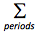(number of parts manufactured within the period)! and becomes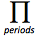(number of parts manufactured within the period)!.

Second, we suggest a new approach to solve this problem. We adapt the principle of small buckets problems to large bucket ones. Small buckets problem assume that at most one product type is manufactured within each period. We then reduce the total number of parts manufactured in the entire horizon by trying to produce all the parts in the earlier periods and thus reducing the frequency of switching from parts.

In section 2, we explain, basing on mathematical models, what differs the CLSPSD from the classical problems namely ULS and CLSP. In section 3, we review the literature about the different problems and the approaches proposed to solve it. In section 4, we describe our approach. In section 5 we study an illustrative example and evaluate the performance of our approach. A conclusion follows.

2. Related Lot sizing models

To explain the difference between the CLSPSD problem and common lot sizing models, we use the mathematical modeling. We detail the ULS followed by the CLSP and small bucket models.

Firstly, we present notation used for expressing sets, decision variables and data commonly used in the models.

Sets

T: set of all periods in the planning horizon indexed by t and m denotes the last period

P: set of all products indexed by i, j, k and l

Decision variables

• Single item problem

xt production level for period t

yt =1 if production occurs at t, 0 otherwise

st inventory level at the end of period t

• Mutli-items problem

xit production level of i in period t

yit =1 if we manufacture product i at t; 0 otherwise

sit inventory level of product i at the end of period t

Parameters

• Single item problem

vct production cost at t

sct set-up cost at t

hct holding cost of product

dt demand due of product at the end of period t

• Multi item problem

vcit production cost of i at t

scit set-up cost of i at t

hcit holding cost of i

dit demand due of product i at the end of period t

capt production capacity of machine at t

vti variable production time consumed to manufacture product i

The single item uncapacitated lot sizing problem (Jans & Degraeve, 2007)

Model (1)(1)(2)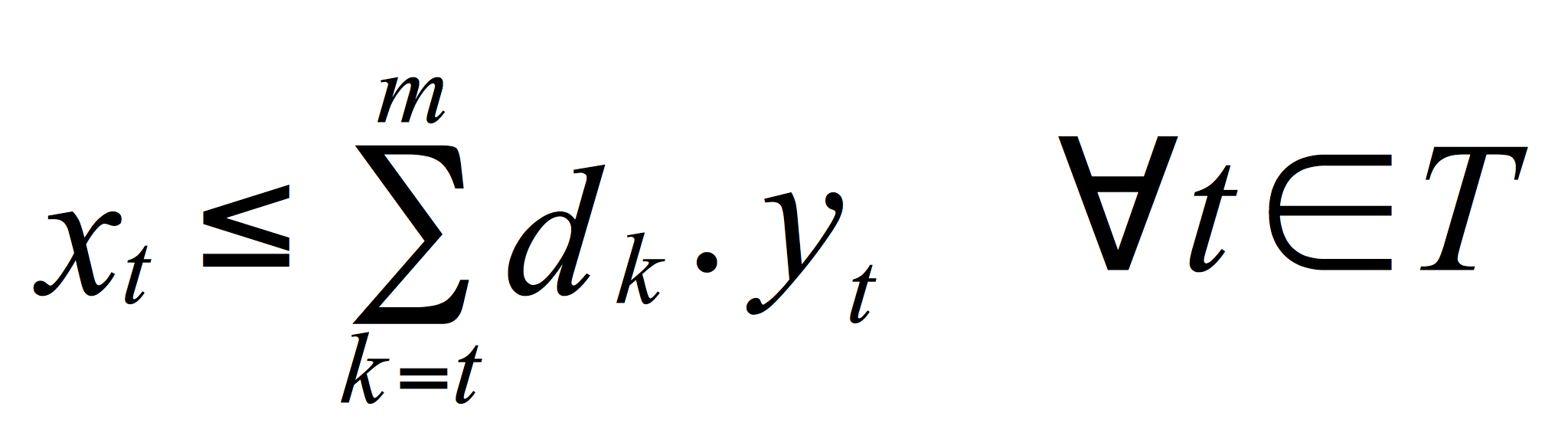(3)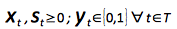(4)

The objective function (1) minimizes the total cost of production, set up and inventory. Constraint (2) is the demand balance equation. Constraint (3) expresses the fact that no ending inventory is allowed, so production is limited by the remaining cumulative demand.

The capacitated lot sizing problem (CLSP) (Jans and Degraeve, 2007)

In this type of problems, many different items can be produced on the same machine in one time period. The machine has a limited production capacity. Thus what differs in the objective function from the previous model is the extra index i used to identify the items.

Model (2)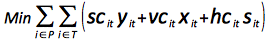(5)(6)(7)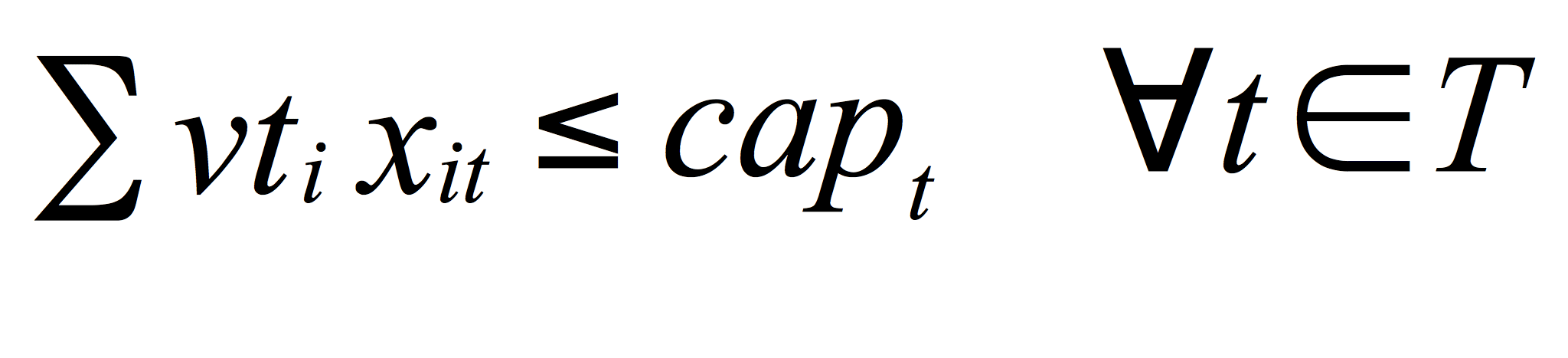(8)(9)

In the set up constraint (7), production is now limited by both the capacity and the remaining demand. Constraint (8) is added to take into account the machine’s capacity.

Small bucket models (Jans & Degraeve, 2007)

In this type of problems, we assume that at most one type of item can be produced on the same machine during each time period.

Model (3)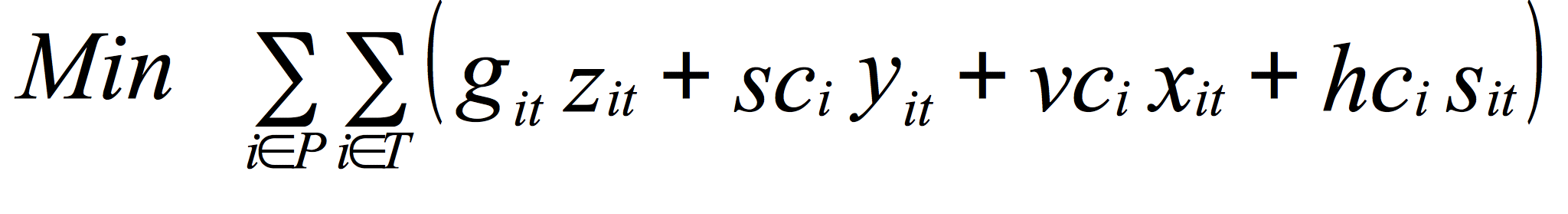(10)(11)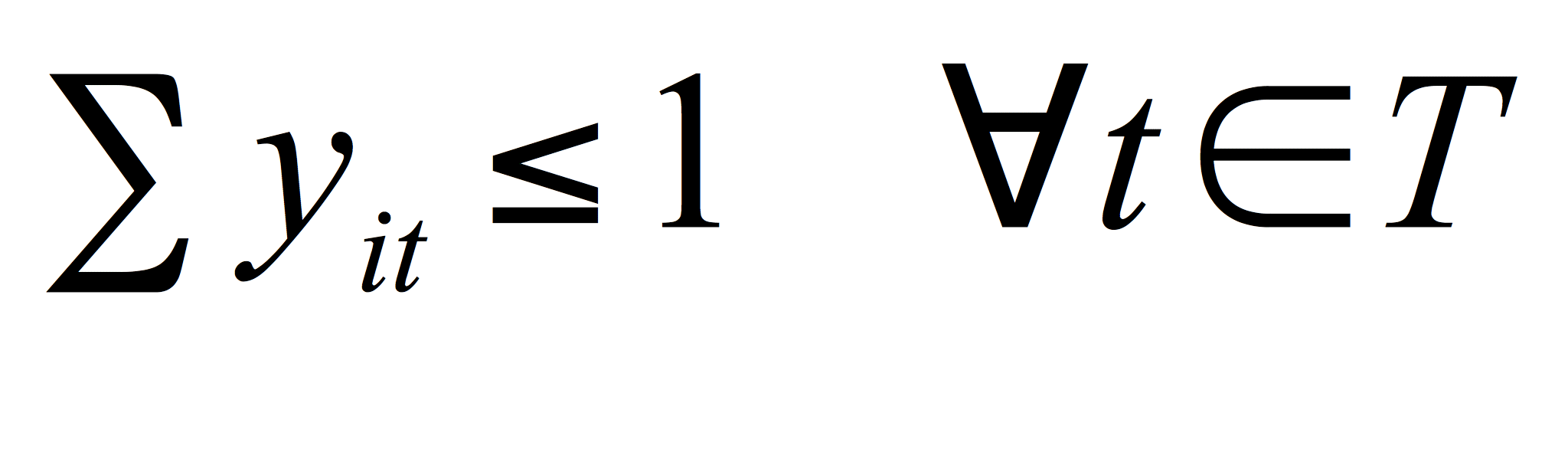(12)(13)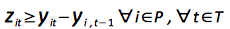(14)(15)

The new variable introduced is the start up variable with an associated start up cost git. A start up occurs when the machine is set up for an item for which there was no set up in the previous period. Constraint (12) illustrates the assumption described before and imposes that the machine can only be set up for at most one item in each period. Constraint (13) allows the production for each item to be up to its capacity if there is a set up. The start up variables are modelled in constraint (14) where zit equals 1 if yit equals 1 and yi t-1 equals 0, thus, if the product i is produced in period t and was not produced in period t-1, a start up occurs.

The capacitated lot sizing problem with sequence-dependent set up times and costs and storage constraints (CLSPSD) (Gupta & Magnusson, 2005)

The model expressed hereafter is the model described by Gupta and Magnusson (2005) using notations of Jans and Degraeve (2007).

Model (4)(16)(17)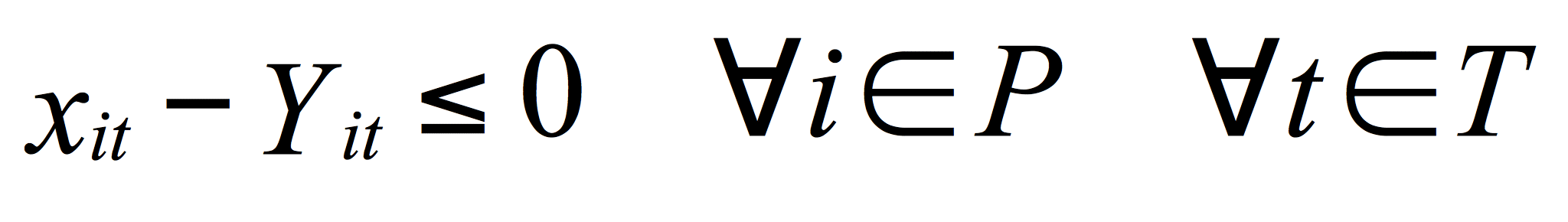(18)(19)(20)

Research by Gupta and Magnusson (2005) assume that machine capacity is normalized to one per period. Production quantities denoted by xit are then denoted in terms of fractions of available capacity. N identifies the number of product types. Yit is a binary variable that equals 1 if product i is produced in period t, 0 otherwise. yijt is a binary variable, equal to 1 if a setup occurs from product i to product j in period t, 0 otherwise. It is assumed that changing over from product i to j takes stij time units of capacity, and variable production costs are ignored.

Constraints (17) and (19) are material balance and capacity constraints, respectively. Constraint (18) ensures that whenever xit > 0, the indicator variable Yit is automatically set to 1.

After describing the CLSPSD problem, we review in section 3 some papers that have treated this problem.

3. Problem definition

3.1. Related works

Many researchers have focused on lot sizing problems but several works have been proposed to solve the CLSPSD problem. Haase and Kimms (2000) study a single-stage, single-machine production system where setup costs and times are sequence dependent. They formulate a large-bucket mixed integer programming which considers only “efficient sequences”. Their work is mainly based on enumerating all possible sequences of products; then, they select those that minimize total setup and holding costs. However, the connection between sequences when switching from a period to another is not considered. This is a serious shortcoming when processing the first product in the sequence of period t+1 after the last product in the sequence of period t is very consuming in setup cost and time. Kovács, Brown and Tarim (2009) extend the work of Haase and Kimms (2000) by developing a dynamic program for computing efficient sequences. They introduce a new mixed-integer programming model in which binary variables indicate whether individual items are produced in a period. Parameters for this program are generated by a heuristic procedure in order to establish a tight formulation. Consequently, they manage to solve problems where the product of items’ number and time periods’ number is at most 60-70, (Kovács et al., 2009).

Meyr (2000) models and solves the problem of integrating lot sizing and scheduling of several products on a single, capacitated production, taking into account sequence-dependent setup times. He determines and schedules continuous lot sizes that meet deterministic dynamic demands and minimize inventory holding costs and sequence-dependent setup costs. He develops a general algorithmic approach where a dual reoptimization algorithm is combined with a local search heuristic. He proves with computational tests the effectiveness of his solution method.

Gupta and Magnusson (2005) study a single machine capacitated lot sizing and scheduling problem with sequence dependent setup costs and non-zero setup times. They provide an exact solution restudied by Almada-Lobo, Oliveira and Carravilla (2008), and a heuristic in order to solve large problem instances. The authors find that their heuristic is more effective when there are many more products than there are planning periods.

3.2. Problem definition

We consider a single production resource with limited capacity. In each time period, multiple products can be manufactured. Backlogging is not allowed. Products’ size is so small that we can store many products and consequently the storage capacity is considered unlimited. Demand is satisfied either by producing in the period or by carrying inventories from earlier periods. Switching from a product to another requires an important setup cost which depends on the sequence and the period. The setup time is negligible and supposed equal to zero.

We have to determine in each period of time, the products to manufacture, their quantities and their sequence. The objective is to minimize the total cost which consists of storage cost, variable production cost, setup and start up cost.

Our contribution concerns both, the problem studied and the approach developed to solve it. We treat a capacitated lot sizing problem with sequence dependent set up costs. Importantly, we consider that setups are incurred not only within but also between periods. To our knowledge, no study has so far dealt with this problem. When we consider setups incurred between periods, a solution like producing (P1-P3-P2) in period 1 and (P4-P5) in period 2 may be excluded if setup cost incurred of producing P4 after P2 is too high.

To solve this type of CLSP, we develop a method that tries to approach the large buckets problem studied here to a small buckets one. Let us explain more this point. Because we consider that more than one product type can be manufactured in each period, we belong to the class Large buckets models. We know that to minimize the total setup costs, we have to manufacture the total demand (over the entire planning horizon) for product i before switching to product j. This approach is the principle of small buckets models: at most, one product type is manufactured in each period.

Though, we do not adopt this restrictive assumption; we just try to approach it by reducing when possible the number of products manufactured within each period.

The principle of small buckets problem provides us a lower bound. We evaluate our solution by using this lower bound and explain under which conditions we can closely approach this lower bound.

3.3. Mathematical formulation

The problem we treat in this paper can be modelled as follows:

Model (5)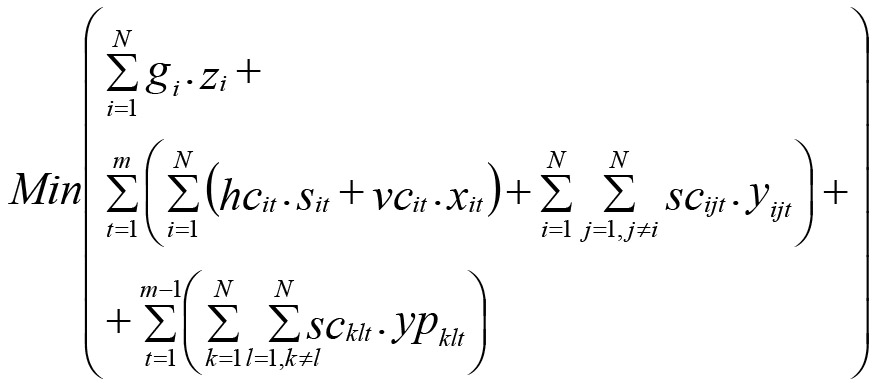(21)(22)(23)(24)(25)

Before giving constraints linking variables xit and both yijt and ypklt, we should note that, in this model, yijt identifies binary variables that correspond to 1 if the product j is manufactured immediately after product i in period t, and 0 otherwise. ypklt identifies binary variables that correspond to 1 if product k is the last product of the production sequence in period t and l the first product of the production sequence in period t+1, and 0 otherwise.

Associated to decision variables sit, xit, yijt, ypklt and zi, binary variables αit and βit are added. αit are Binary variables that correspond to 1 if product i is manufactured in period t and 0 otherwise. βit are Integer variables that indicate the position of product i in the production sequence, at period t. Let us give and then explain constraints between this set of variables.(26)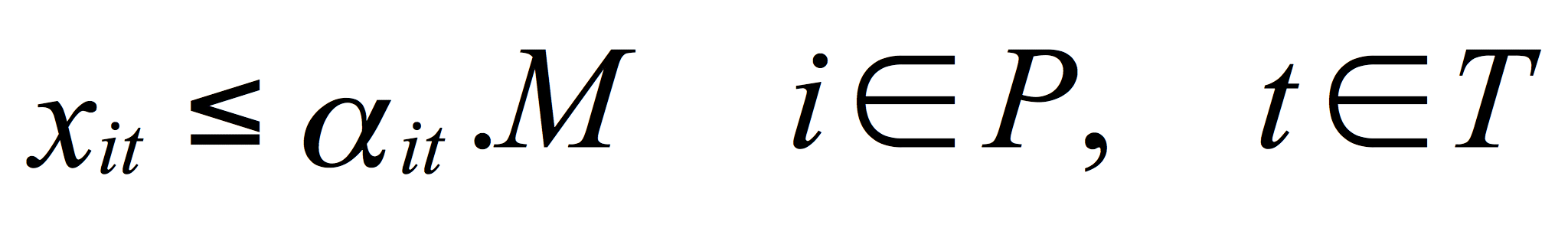(27)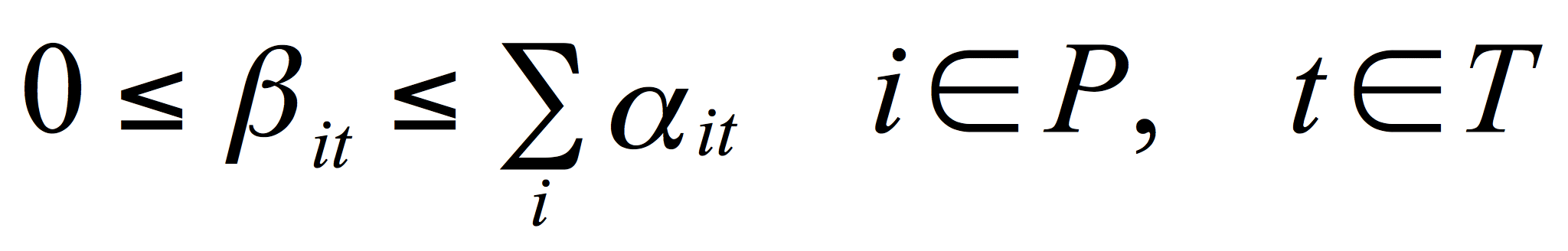(28)(29)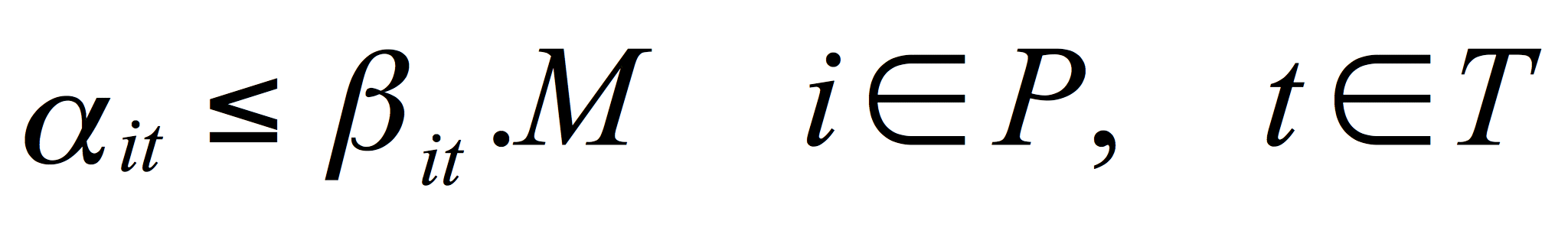(30)(31)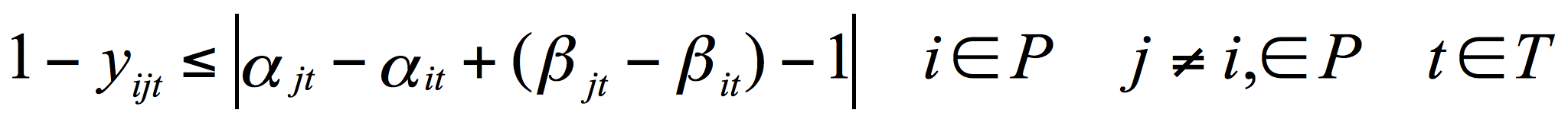(32)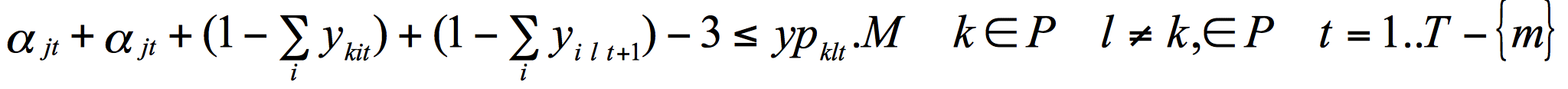(33)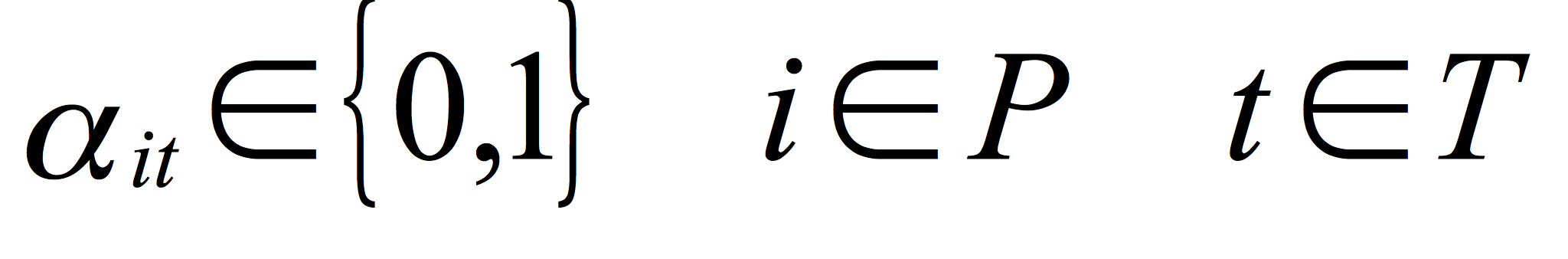(34)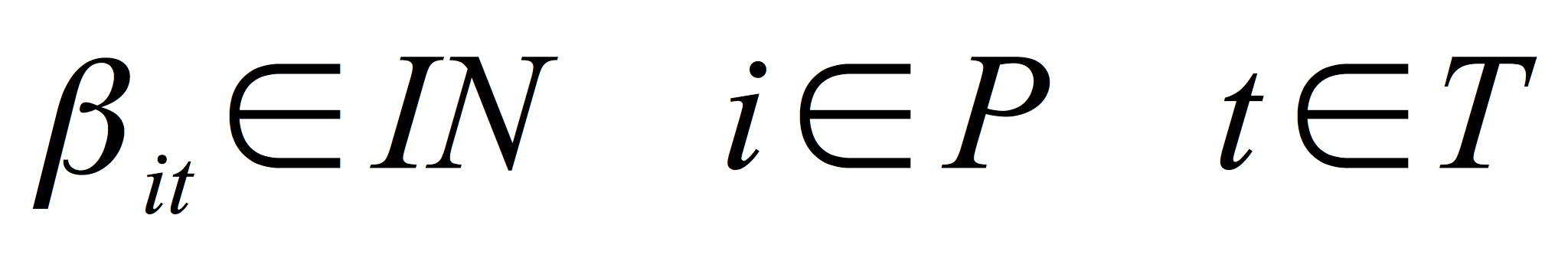(35)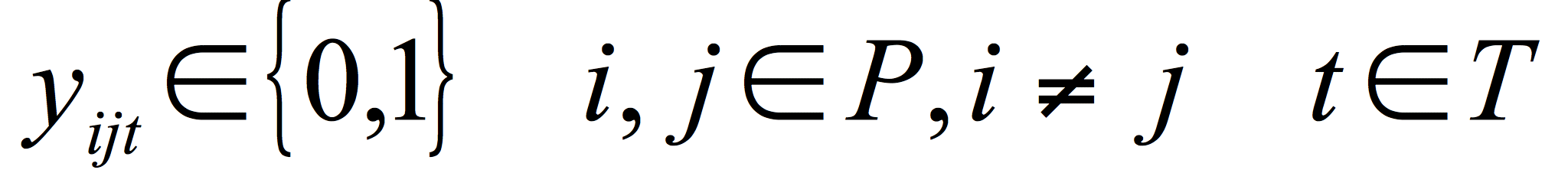(36)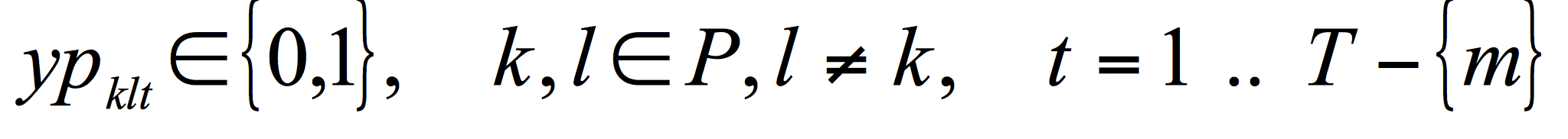(37)

Constraints (26) and (27) indicate that if we manufacture product i in period t then variables αit equal 1, thus, if amounts xit are strictly positive then αit equal 1. Relation between variables αit and βit are determined by constraints (28), (29) and (30). While constraints (29) and (30) affect 0 to βit when product i is not manufactured in period t, i.e. when αit equals 0, constraints (28) determine their domains of variation. These domains are delimited by 0 and the number of products manufactured at period t, i.e. the sum of αit. Constraints (31) avoid attributing the same position in the production sequence to two different items. In fact, if we manufacture products i and j at period t, i.e. if αit and αjt equal 1, then (βjtβit) is necessary greater than 1 or lower than (-1). Constraints (32) attribute the value 1 to variables yijt when products i and j are manufactured at period t (βit and βjt equal 1) and item’s j position succeeds item’s i position in the production sequence, what means product j is manufactured immediately after product i (βjtβit =1). Finally constraints (33) imply that if product k has the last position in the production sequence at period t and product l has the first position in the production sequence at period (t+1) then the binary variable ypklt equals 1. The last product of the production sequence at period t verifieswhat means no product is manufactured after product k, in the period t. The first product of the production sequence at period (t+1) verifieswhat means only one product is manufactured after product l, in the period (t+1).

Finally, gi denotes start up cost associated to manufacturing product i and zi binary variable that corresponds to 1 if product i is the first product manufactured in period 1 and 0 otherwise. Start up costs concern only period 1 and are considered in the following constraints.(38)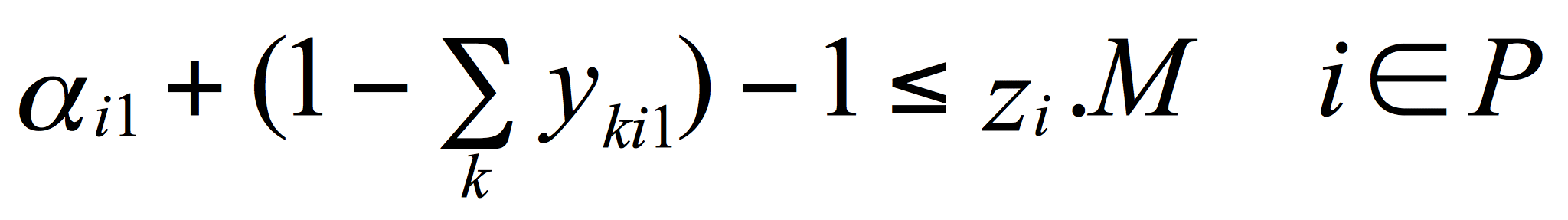(39)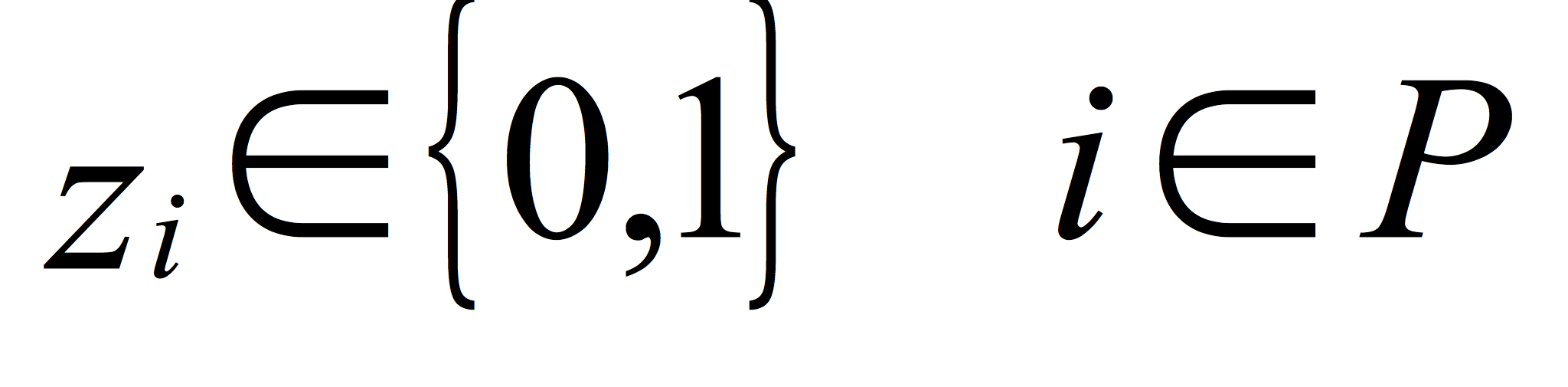(40)

Constraints (38) assign the value 0 to zi if product i is not produced in the first period and constraints (39) assign the value 1 to zi if product i is manufactured in the first period (αi1=1), and this product is the first product manufactured at this period, this means that no product precedes i, so.

The difference between our model and Gupta’s model is the binary variables introduced in the objective function to express costs incurred from switching from one period to another.

This NP Hard problem is modelled as a non linear mathematical model, (Chaieb Memmi & Hammami Laaroussi, 2010). To solve it, we propose the approach described Section 4.

4. Proposed Approach

We propose in this paper a new approach for solving a CLSPSD problem. The main idea is to reduce the number of products to manufacture within each period in order to decrease the setup cost.

The approach is composed of three steps. First, we propose to compute a lower bound on total cost. This lower bound is given by adding the inventory and production costs determined without considering the setup and start up costs; and the setup and start up costs determined by relaxing the constraint of limited capacity. Determining this lower bound is detailed in the first step. Then we propose a three sub-steps iteration procedure. Firstly, we solve optimally the lot sizing problem without considering products sequencing and their cost. Thus, we determine products quantities to produce each period while minimizing the storage and variable production costs. Given the products to manufacture each period, we determine its correspondent optimal products sequencing, by using on a B&B algorithm. Given the sequences of products within each period, we evaluate the total start up and setup cost. The total cost of this solution is the sum of the cost of lot sizing problem and the start up and setup cost. We compare then the total cost obtained, to the lower bound of the total cost. If this value riches a prefixed value, we stop. Otherwise, we modify the results of lot sizing problem by assigning to the lowest economic amountcorresponding to the pair (product i1, period t1), the value 0 (see equation. (41)). We resolve the lot sizing problem after inserting the new constraint. If there is no feasible solution, we update the set E (E=E-{(i1,t1)}) and we assign the value 0 to another economic product Qi2t2. We repeat determining products sequencing within periods, evaluating the criterion’s value and comparing it to a prefixed value until the prefixed value is reached or after an important number of iterations without ameliorating the criterion’s value.(41)

4.1. Step 1, Lower Bound determination

We recall that the total cost we aim to minimize is composed of:

• Production and holding costs

• Setup and start up costs

In order to determine a lower bound to the total cost, we compute a lower bound for its components, Production and Holding costs, and Setup and start up costs.

For the first component, we model the lot sizing problem without considering setup and start up costs. The model obtained is linear and is described below.

Model (6)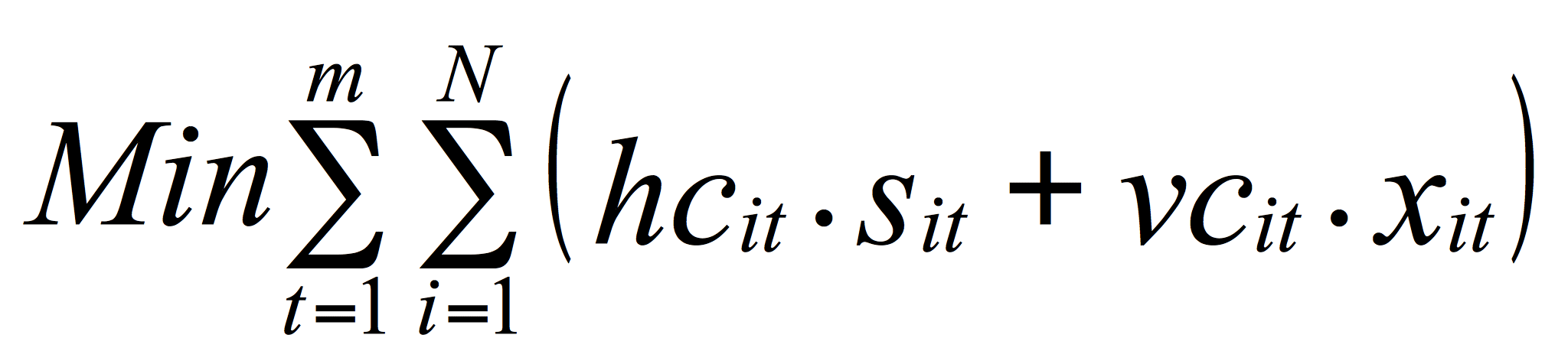(42)(43)(44)(45)

Constraint (43) is the demand balance equation and (44) expresses that the production is limited by the machine’s capacity.

When solving this linear model, we obtain:

• The optimal Production and Inventory cost, let ZL denotes this cost.

• The optimal products quantities to manufacture each period. Given these amounts we are able to set in the set of parts to manufacture each period. Assume, for example, three product types. In period 1, the amounts of products P1 and P2 are different from zero whereas the amount of products P3 equals zero. We know that the set of parts that will be manufactured in period 1 is {P1, P2}. We need then to find whether we should manufacture P1 before P2 or P2 before P1.

To determine the lower bound for setup and start up costs, we relax the machine capacity constraint. We suppose, if we are manufacturing product type i, that we do not switch for another product type before producing the total demand (over the entire planning horizon) of product i. This approaches the principle of Small buckets models which assume that at most one product type is manufactured within each period.

Therefore, to determine the lower bound of setup and start up costs, the problem consists in simply enumerating all the possible sequences of products, each sequence engender a total setup and start up cost. We then retain the sequence that minimizes this total cost, this total cost constitutes the lower bound we searched for. If we have to manufacture three types of products (P1, P2, P3), there are six possible sequences {P1-P2-P3}{P1-P3-P2}{P2-P1-P3}{P2-P3-P1} {P3-P1-P2}{P3-P2-P1}. Total cost incurred by the sequence {P1-P2-P3} is given by: Start up cost (P1)+setup cots (P1 to P2)+ setup cots (P2 to P3)

The lower bound LB is the sum of lower bound of production and inventory cost, zL*, and the lower bound of start up and setup cost zs*. The algorithm for computing LB is given in Appendix A.

4.2. Step2, Iterative procedure for finding a feasible solution

As we mentioned earlier, the step consists of three sub-steps, cf. Appendix A.

4.2.1. Sub-Step2.1 Production plan determination

Firstly, we solve optimally the lot sizing problem (Model 6) without considering products’ sequencing and their cost. Thus, we determine quantities to produce each period while minimizing the storage and variable production costs.

4.2.2. Sub-Step2.2 B&B algorithm for solving the sequencing problem

Since the products to manufacture are already determined in the first step, it remains to schedule the products within each time period in order to minimize start up and setup costs. Scheduling separately each time period regardless of the setup costs between periods can degrade the solution’s performance. That is why we have to schedule the products with consideration to the costs incurred from switching from one period to another. We proposed to solve the sequencing problem with a branch and bound method motivated by its practical advantage: finding an optimal solution by reducing the search space.

We illustrate the sequencing problem with a simple example. Suppose that the amounts provided by solving the lot sizing problem (without considering start up and setup costs) provides for three part types and over three periods the following amounts:

• Period 1: amounts of (P1,P2,P3)=(100,200,0)

• Period 2: amounts of (P1,P2,P3)=(100,200,300)

• Period 3: amounts of (P1,P2,P3)=(0,400,100)

Consequently, the set of parts to schedule for respectively periods 1, 2 and 3 are {P1,P2}{P1,P2,P3}{P2,P3}.

The solution of the sequencing problem has the same form. In fact, we will obtain these sets of parts. What will differ is just the schedule of parts within each set.

In what follows we describe after recalling some principles of the B&B method, the algorithm we have developed.

A branch and bound method B&B enables to enumerate all solutions of search space to find the optimal one, Pessan, Bouquard and Néron (2008). The search space in a B&B method is represented by a tree stored using a data structure containing the nodes that have not yet been explored.

The algorithm consists of three main components: (1) a bounding function, (2) a strategy for selecting and (3) a branching rule, (Clausen & Perregaard, 1999). There are two standard ways to calculate the lower bound function: maintain the objective function but relax the sub-problem or maintain the feasible region but modify the objective function. We distinguish three strategies for selecting, (1) the best first search strategy BeFS, (2) the breath first strategy and (3) the Depth First Strategy DFS. The BeFS consists of selecting among the live sub-problems the lowest bound node. The breath first strategy corresponds to a situation where all nodes at one level are treated before any node at a higher level. In a DFS, we choose the node with the largest level to explore. A breadth-first search strategy requires less memory than the best-first strategy, (Zhou & Hansen, 2006). The depth first strategy has the advantage of finding feasible solution quickly and keeping a reasonable stack size, Pessan et al. (2008). Finally, the decision “how to separate” depends on the speed to find a new sub-problem.

In addition to the three components, we need to compute an initial solution enabling us to have an upper bound. The objective value of the initial solution represents the upper bound on the objective function. Searching an initial good feasible solution facilitates fathoming of nodes as early as possible, (Clausen & Perregaard, 1999).

The B&B algorithm we developed consists of three main procedures:

Procedure 1: Computing an initial solution and determining an Upper Bound

To determine an initial solution for our sequencing problem, we proceeds by searching from the set of parts to manufacture on period 1, the part Pi that has the lowest start up cost. We then assign to this part the first position in the set. In the second position, we choose the part Pj that provides the lowest set up cost incurred when switching from Pi to Pj. We continue this processing over the next time periods. Suppose that Pk is the last part to manufacture in period t, we choose from the set of parts to manufacture in period t+1 the part Pl that implies the least setup cost with Pk.

Procedure 2: Computing the lower bound

It is well known that the quality of the lower bound is one of the most critical elements of any branch-and-bound algorithm. As we mentioned above, there are two ways to obtain the lower bound. In most cases, computing a lower bound consists of relaxing some constraints (in different ways) and in solving a new easier problem.

In our algorithm, we consider one main relaxation to obtain lower bounds. For all products not yet scheduled, we relax the constraint of respecting the demand. In other words, we have the possibility not to schedule the remained products. The lower bound LB we consider is the start up and setup costs incurred by the scheduled parts to which we add the minimum setup cost multiplied by the number of remained positions to fill. To illustrate, consider again the example of scheduling {P1,P2}{P1,P2,P3}{P2,P3}. Suppose that we have already scheduled P1, P2 in period 1 and assigned the first position to P1 in period 2, so we have for example {P2-P1}{P1-?-?}{?-?}, it remains to schedule four products; the lower bound is given by:

{start up cost(P2) + setup cost(P2-P1) + setup cost(P1-P1) +
(number of parts that remain to schedule-1)*Min setup cost different from zero}

Procedure 3: Branching scheme and search strategy

The branching scheme consists of scheduling a new product after a partial schedule.

For the previous example, {P2-P1}{P1-?-?}{?-?} constitutes a partial schedule.

We select the product that is not yet scheduled and that maximizes the lower bound. As the search space is explored by using the depth first strategy, if the value of such a lower bound is greater than the value of the upper bound, then this node is removed.

We need to compute the lower bound of each node included in the tree. A node included corresponds to a product selected and scheduled in the first available case. For each case already scheduled, we have as information: the product selected to schedule, products already scheduled, the lower bound reached, and the current time period.

This algorithm is described in Appendix A.

4.2.3. Sub-Step2.3 Performance evaluation of a feasible solution

We compute total cost of feasible solution obtained. It is the sum of production and inventory costs, computed in sub-step1, and the startup and setup costs computed in sub-step2. If the difference between the total cost and the lower bound, calculated in step1, riches a prefixed value; or the prefixed maximum number of iterations is reached, the algorithm stops. Otherwise we modify the results of lot sizing problem by inserting a new constraint which implies to assign the value 0 to the lowest economic amount. We then return to substep1 and we resolve the lot sizing problem after inserting the new constraint.

5. Computational results

Our approach has important practical implications. It determines the products to manufacture each time period, their economic amounts, and their scheduling within each period. This outcome should help decision makers bearing expensive start up and setup costs, to reduce their Inventory and Production costs and Start up and setup cots.

In this section we evaluate the performance of our approach and assess its limits. We study three plausible scenarios with an illustrative example. In the first one, we choose start up and setup cost significantly higher than production and inventory costs. In the second scenario, we study the effect of varying machine’s capacity on results and approach’s performance. In the third scenario, we show that the performance reached in first and second scenarios is noticeably moderated when production and inventory costs are important.

We describe in what follows, the Illustrative example. We consider a planning horizon composed of four periods and four product types to manufacture on a single capacitated machine. We assume the following data that reveals start up and setup costs are more important than production and inventory costs:

• Average Inventory cost multiplied by average demand = 1% average setup cost

• Average Setup cost = 10% average start-up cost

• Average production cost multiplied by average demand = 10% setup cost

• Machine capacity = number of items multiplied by (3/2 x Maximum demand)

Among the set of equivalent demands, some demands are much more inconsequential.

Inventory, production, start up and setup costs, processing times and demands are presented in Appendix B.

Scenario 1: Evaluating the approach’s performance and Comparing between the developed approach and the traditional sequential approaches

Usually, when solving the lot sizing problems, parts are not scheduled within periods and thus, setup costs are not considered. The sequences of items are determined independently and without re-examining the results of the lot sizing problems. In our works we modify these results (by assigning to some of them the value 0 as explained section 4). Table 1 shows that we manage to reduce the total cost, determined by the traditional approach, by 30%.

 Solution Lot sizing cost “ZL” Start up and setup costs “ZS” Total cost “TC” Traditional approach 54,4 2700 2754,4 Our approach 94,4 1850 1944,4 Gap 73,53% -31,48% -29,41%

Table 1. Comparing the New approach with the Traditional one

Our developed approach has improved the traditional approach by about 30% in terms of total cost. To explain the reduction in total cost incurred by our approach, we compare the economic amounts xit, the inventory level sit and the sequences of items provided by the two approaches and illustrated in Table 2.

 Solution Traditional approach New approach Economic amount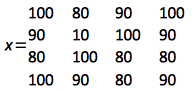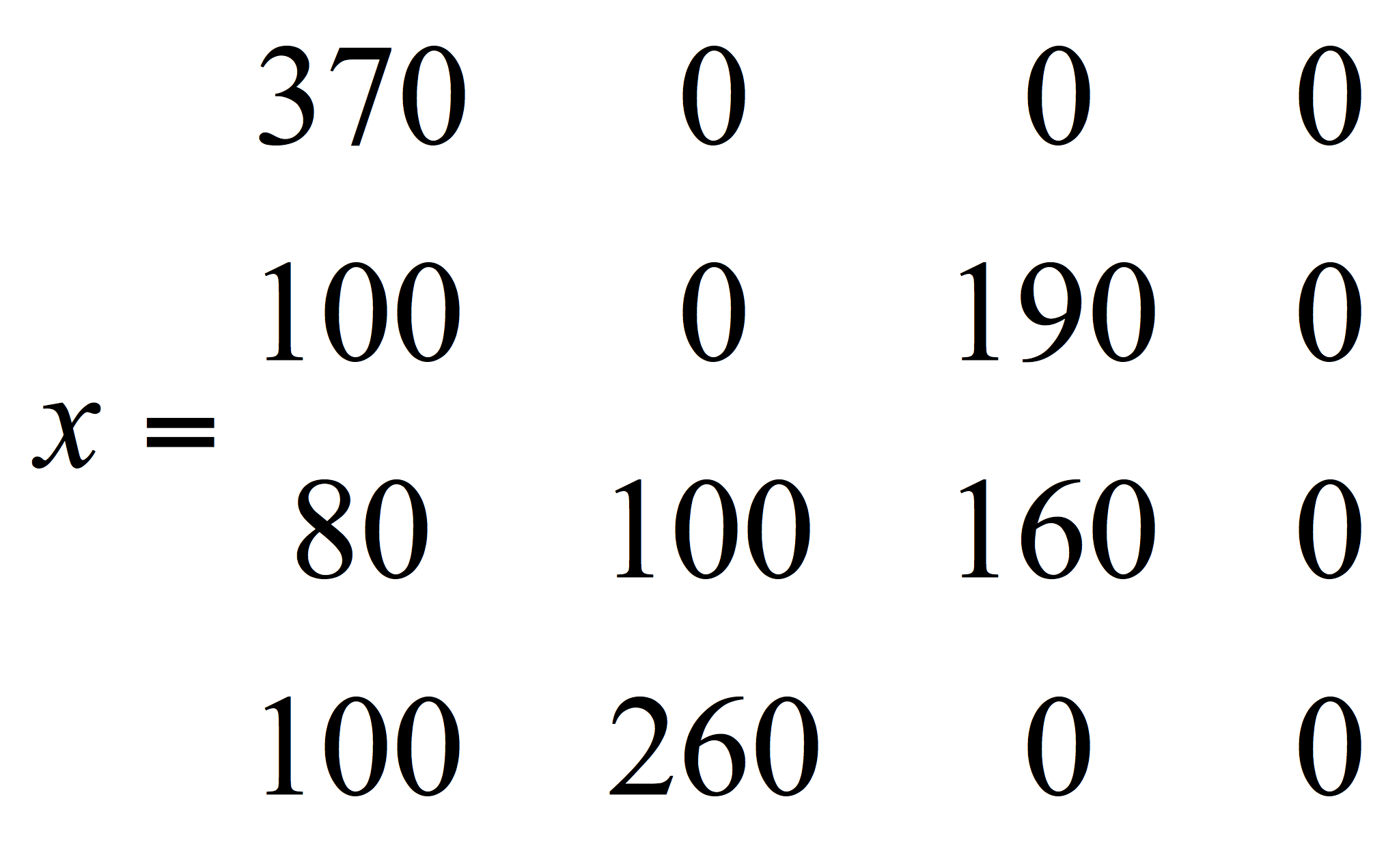Inventory level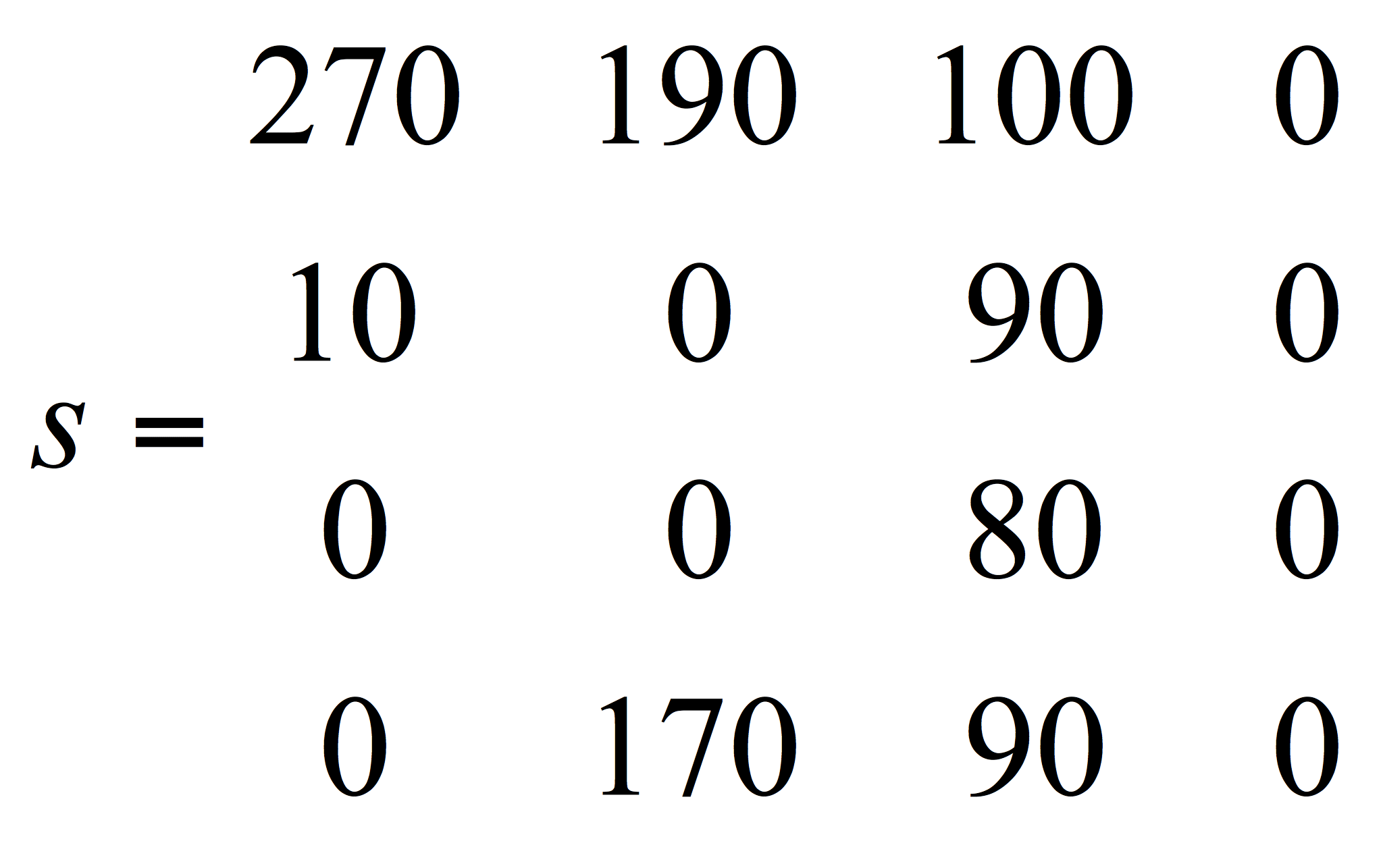Sequence 1243 3124 1243 3124 1324 43 32

Table 2. Comparing Economic amounts, Inventory levels and Sequences

As we can see in Table 2, we have succeeded to reduce the number of setups. The number of type products manufactured in period 2 is reduced from 4 given by the traditional approach to 2. In period 3, the number is reduced from 4 part types to 2 and in period 4, from 4 to 0. This is derived from the economic amounts matrix where we have eight amounts equal to zero. However, in the traditional approach, none of the amounts equals zero. In fact, the machine capacity and storage capacity allow manufacturing the products earlier to store them, and to satisfy demand of following periods. After iteration 8 (Figure 1), we cannot increase anymore the number of zeros in economic amounts matrix otherwise the machine capacity will be exceeded.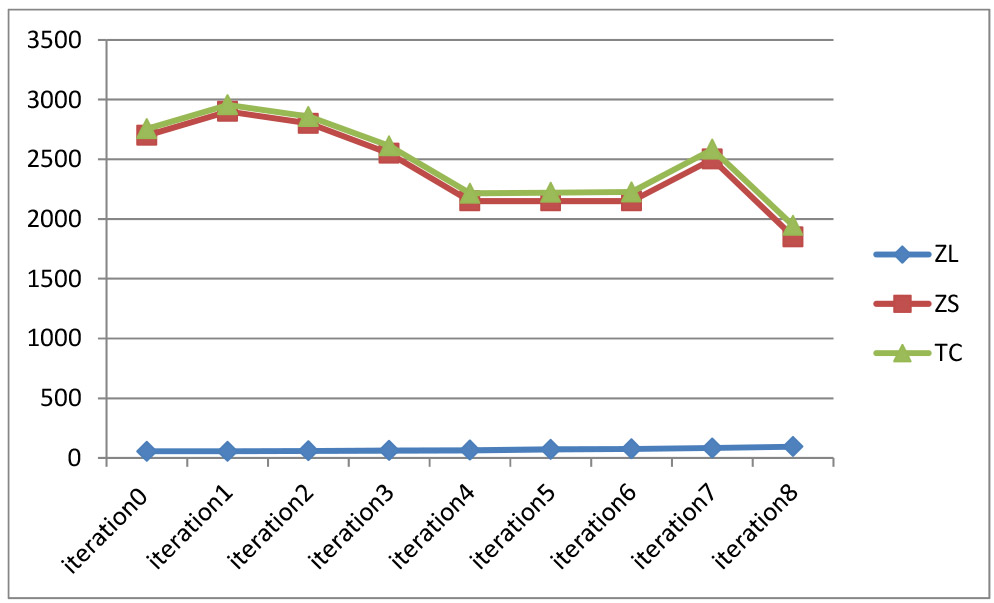Figure 1. Total cost, Lot sizing cost (ZL) and Scheduling cost (ZS) obtained when applying the proposed approach

When the inventory cost is not so important, clearly the decision maker regroups the products in earlier periods to reduce the frequency of switching from one part type to another. Thus, the total number of parts produced over the planning horizon is also reduced. Due to machine capacity constraint, grouping all products to manufacture in earlier periods is not usually possible. Besides, notice that, when machine capacity is limited, reducing the total number of parts (to manufacture over the entire planning horizon) could increase set up costs. We observe this case in iteration 7. The total number of products manufactured decreased, while the total start up and setup cost increased. In fact, in iteration 6, we were manufacturing ten products; the total start up and setup cost were equal to 2150 mu. This number decreased to nine products whereas the start up and setup costs increased by 16%. The reasons to this increasing are:

First, the triangular inequality, setupcost[Pi, P j] < setupcost[Pi, Pk]+ setupcost[Pk, Pj]

is not usually verified, this is the case hereafter:

setupcost[P2,P1](650)> setupcost[P2,P4](250) + setupcost[P4,P1](50)

Second, switching from period (t) to period (t+1) may deteriorate the total setup cost. Suppose for example, that the last product we manufacture in period t is Pi and when reducing the total number of parts, we eliminate Pi from the set of parts to manufacture in period t+1. Any part that has the first position in the sequence of period (t+1) will engender greater set up cost than when we keep producing the same part type Pi.

Scenario 2: Comparing the approach’s results with the Lower Bound

In this scenario, we aim to compare results of our approach to the lower bound described in Section 4. This lower bound on total cost is composed of (1) lower bound on production and inventory cost (that is lower bound on lot sizing cost), and (2) the lower bound on the start up and setup cost (lower bound for the scheduling problem).

Computing the lower bound for this illustrative example, we find:

• Lower bound on lot sizing cost = 54,4 mu

• Lower bound on start up and setup cost = 750 mu, it corresponds to the sequence (1‑2-4-3)

• Lower bound on total cost = 54,4+750= 804,4 mu

In this example, the total cost according to the traditional approach’s solution is 2754.4 which represent 250% of the lower bound, whereas, the total cost of our approach’s solution is 1944,4 which represents 150% of the lower Bound (iteration8). This gap can be improved if we increase the machine’s capacity to 2*maximum demand*number of items; it becomes equal to 90% (iteration 10), and if the machine capacity reaches 4*maximum demand*number of items, the gap is reduced to 10% (iteration 12).

We present in Figure 2, Figure 3, Figure. 4, respectively, the evolution of lot sizing cost, start up and setup cost, total cost and their lower bounds. Figure 2 shows that the lot sizing cost increases with the number of iterations. Because, we produce all parts in earlier periods, inventory costs are systematically increased. Figure 3 shows that start up and setup cost reaches the lower bound in the last iteration where we do not switch for a new part type if we have not produced the total demand for the current product.

Here, since start up and set up costs are much more important than production and inventory costs; the total cost is too close to its lower bound (the gap is 10%), Figure 4.Figure 2. Lot sizing cost and its lower boundFigure 3. Scheduling cost and its lower bound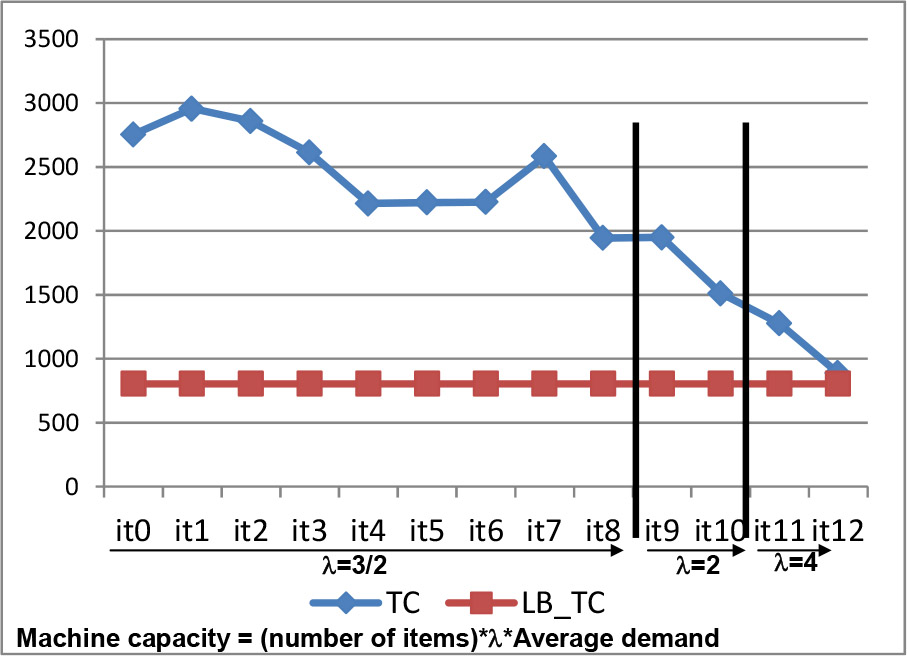Figure 4. Total cost and its lower bound

Scenario 3: Limits of the approach

Our approach provides interesting results when the inventory costs are not very important (this is the case in scenario1) and the machine capacity is not limiting (as explained in scenario2). Under these conditions, the total cost follows the evolution of setup and start-up cost (Figure 5). This scenario illustrates that decreasing the total cost is considerably moderated when production and inventory costs are too important. This is shown in Figure 5 where the average unitary production cost multiplied by the average demand equals the average setup cost, the average unitary inventory cost multiplied by the average demand, equals 1%, 10% and then 100% of average setup cost. In the first case, the total cost decreases when start up and setup costs decrease. In the second, we manage to decrease the total cost even though the inventory costs are important. In case 3, the reduction in total cost is insignificant despite the decrease in the start up and setup costs.

Notice that for the three scenarios more than 90% of demands are close to the maximum demand (please refer to the matrix of demand in Appendix B). Consequently, when we assign the value 0 to one of the amounts resulting from solving the lot sizing problem, the impact on its cost is very important. Nevertheless, we manage to considerably reduce the total cost. This is the case especially when Production and Inventory costs are not too high.Figure 5. Effect of varying inventory cost

6. Concluding remarks

In this paper, we propose an iterative approach for solving a lot sizing and scheduling problem with sequence dependent setup costs. Our model explicitly accounts for the setup costs between periods planning and fits better to real-world situations where production systems run continuously. The key element of our approach is to reduce the number of items to manufacture over the entire planning horizon. Therefore, the frequency of setting up the production machine is also reduced.

In the case of limited machine’s capacity, we show that setup costs could increase when reducing the total number of parts manufactured over the entire planning horizon. In fact, the triangular inequality in setup costs is not usually available, as shown in scenario 1. We can compute a lower bound to setup costs if the total number of manufactured part is reduced to the strict number of part types. To this lower bound, we add the lower bound of Production and Inventory costs.

We illustrate the economic significance of our result using a 4 periods and 4 products case. We obtain a deviation of 10% from the lower bound. This deviation depends on the machine’s capacity and on the significance of Inventory and Production costs. Similar to the heuristics proposed in the literature, the performance of our iterative approach is data-dependent. Rather, we view it as a tool that offers valuable guidance to decision makers in manufacturing facilities. Further research on modeling setup times is needed.

Acknowledgements

The authors would like to acknowledge G. Manita for his support in computer implementation.

References

Allahveverdi, A., & Soroush, H.M. (2008). The significance of reducing setup times/setup costs. European Journal of Operational Research, 187(3), 978-984. http://dx.doi.org/10.1016/j.ejor.2006.09.010

Almada-Lobo, B., Oliveira, J.F., & Carravilla, M.A. (2008). The capacitated lot-sizing and scheduling problem with sequence-dependent setup costs and setup times. Computers and Operations Research, 35(4), 1374-1376. http://dx.doi.org/10.1016/j.cor.2006.08.019

Belvaux, G., & Wolsey, L.A. (2000). bc—prod: A Specialized Branch-and-Cut System for Lot-Sizing Problems. Management Science, 46(5), 724-738. http://dx.doi.org/10.1287/mnsc.46.5.724.12048

Clausen, J., & Perregaard, M. (1999). On the best search strategy in parallel branch-and-bound: Best-First Search versus Lazy Depth-First Search. Annals of Operations Research, 90(0), 1-17. http://dx.doi.org/10.1023/A:1018952429396

Chaieb Memmi, I., Hammami Laaroussi, S. (2010). A new formulation for a variant of the Capacitated Lot Sizing and Scheduling Problem with Sequence Dependent setup costs and times: Single machine, multiple products and periods. Proceedings of the 5th IFAC International Conference on Management and Control of Production and Logistics, MCPL 2010, Coimbra, Portugal, 83-88.

Gupta, D., & Magnusson, T. (2005). The capacitated lot-sizing and scheduling problem with sequence-dependent setup costs and setup times. Computers and Operations Research, 32(4), 727-747. http://dx.doi.org/10.1016/j.cor.2003.08.014

Haase, K., & Kimms, A. (2000). Lot sizing and scheduling with sequence-dependent setup costs and times and efficient rescheduling opportunities. International Journal of Production Economics, 66(2), 159-169. http://dx.doi.org/10.1016/S0925-5273(99)00119-X

Jans, R., & Degraeve, Z. (2007). Meta-heuristics for dynamic lot sizing: A review and comparison of solution approaches. European Journal of Operational Research, 177(3), 1855‑1875. http://dx.doi.org/10.1016/j.ejor.2005.12.008

Karimi, B., Fatemi Ghomi, S.M.T., & Wilson, J.M. (2003). The capacitated lot sizing problem: a review of models and algorithms. OMEGA The International Journal of Management Science, 31(5), 365-378. http://dx.doi.org/10.1016/S0305-0483(03)00059-8

Kolfer, M., Wagner, S., Beham, A., Kronberger, G., & Affeenzeller, M. (2009). Priority Rule Generation with a Genetic Algorithm to Minimize Sequence Dependent Setup Costs. Computer Aided Systems Theory - EUROCAST 2009. Lecture Notes in Computer Science, 5717, 817-824.

Kovács, A.,N., Brown, K., & Tarim, S.A. (2009). An efficient MIP model the capacitated lot-sizing and scheduling problem with sequence-dependent setups. International Journal of Production Economics, 118(1), 282-291. http://dx.doi.org/10.1016/j.ijpe.2008.08.033

Meyr, H. (2000). Simultaneous lotsizing and scheduling by combining local search with dual reoptimization. European Journal of Operational Research, 120(2), 311-326. http://dx.doi.org/10.1016/S0377-2217(99)00159-9

Pessan, C., Bouquard, J.L., & Néron, E. (2008). Genetic Branch-and-Bound or Exact Genetic Algorithm. Artificial Evolution. Lecture Notes in Computer Science, 4926, 136-147. http://dx.doi.org/10.1007/978-3-540-79305-2_12

Ullah, H., & Parveen, S. (2010). A literature Review on Inventory Lot Sizing Problems. Global Journal of Researches in Engineering, 10(5), 21-36.

Zhou, R., & Hansen, E. (2006). Breadth-first heuristic search. Artificial Intelligence, 170(4-5), 385-408. http://dx.doi.org/10.1016/j.artint.2005.12.002

Appendix A. Iterative approach for solving the CLSPSD problem